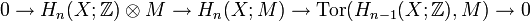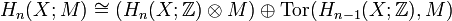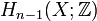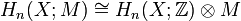# Universal coefficient theorem for homology

Jump to: navigation, search
For an algebraic version of the theorem, see Groupprops:Universal coefficient theorem for group homology

## Statement

### For coefficients in an abelian group

Suppose$M$ is an abelian group and$X$ is a topological space. The universal coefficients theorem relates the homology groups for$X$ with integral coefficients (i.e., with coefficients in$\mathbb{Z}$) to the homology groups with coefficients in$M$.

The theorem comes in two parts:

First, it states that there is a natural short exact sequence:$\! 0 \to H_n(X; \mathbb{Z}) \otimes M \to H_n(X;M) \to \operatorname{Tor}(H_{n-1}(X;\mathbb{Z}),M) \to 0$

Second, it states that this short exact sequence splits, so we obtain:$H_n(X;M) \cong (H_n(X;\mathbb{Z}) \otimes M) \oplus \operatorname{Tor}(H_{n-1}(X;\mathbb{Z}),M)$

### For coefficients in a module over a principal ideal domain

Fill this in later

## Particular cases

### Case of free abelian groups

If$H_{n-1}(X;\mathbb{Z})$ is a free abelian group, then we get:$H_n(X;M) \cong H_n(X;\mathbb{Z}) \otimes M$

As a corollary, if all the homology groups are free abelian, then the above holds for all$n$.